# A geometric model that has a maximum speed

## Abstract

Introduce a geometric model in which there is a maximum speed, which may help us understand why the speed of light c is the maximum speed in the universe, why parameter c is a constant and the geometric mechanism that causes time dilation and length contraction. Finally, this paper will introduce a new coordinate transformation and general velocity addition formula based on this model. The new coordinate transformation and general velocity addition formula not only correspond to time dilation/length contraction effect, but also correspond to the Sagnac effect.
Keywords: Lorentz transformation, special relativity, one way speed of light, Sagnac effect

## Introduction

The speed of light c in vacuum has a very important position in physics, which is the basis of special relativity. The traditional geometric model of special relativity is Minkowski (-+++) space, Hans Montanus discussed the possibility of using an absolute Euclidean (++++) space-times as a model for special relativity too. This article will introduces an absolute Euclidean geometric model for the maximum speed instead of geometric model for Lorentz transformation, which will explain why the speed of light c is the maximum speed, why parameter c is a constant, and the geometric mechanism that causes time dilation and length contraction. Finally, this paper will introduce a new coordinate transformation and general velocity addition formula based on this model. The new coordinate transformation and general velocity addition formula not only correspond to time dilation/length contraction effect, but also correspond to the Sagnac effect.

## Geometric model

There is no definition of abstract dimensions in classical geometry, so when discussing the interaction between four-dimensional object and three-dimensional space, we believe that four-dimensional object can move out of three-dimensional space. By use a virtual abstract four-dimensional space instead of a real four-dimensional space to define four-dimensional object, I would like to propose four-dimensional object and three-dimensional space always have an intersection point when there is only four-dimensional object and three-dimensional space.
This article will borrow the idea from object-oriented programming to define the abstract dimension.

Definition of abstract spatial dimension and instantiation of abstract spatial dimension:
1. In geometry, we can define a real axis as an abstract spatial dimension.
2. Once an abstract spatial dimension is instantiated by a physical entity like physical space or object, the abstract spatial dimension becomes a physical spatial dimension.
3. When abstract spatial dimension is not instantiated, abstract spatial dimension is merely a parameter in thought process; we should set all parts that are not instantiated as 0.

Reasoning:
1: In abstract space, the sum of the same-directional length (L(A->B)+L(B->A)) of any two points (A, B) on a dimension is equal to the sum of the lengths of all entities on this dimension.
2: According to the reasoning A, four-dimensional object should be isotropic (connect itself end to end) in the fourth abstract dimension if there is only a three dimensional real space, four-dimensional object cannot move out of the three dimensional real space but always have an intersection point with three dimensional real space.

Figure 1. Compare the process of a four-dimensional object pass through a three-dimensional real space, classical geometry (above) vs. this article (below).

Let's setup a procedure to discuss the interaction of four-dimensional object and three-dimensional real space.

1. Set up a virtual abstract four-dimensional Euclidean space with axis X, Y, Z, and W.

2. If the coordinate points of an object in the virtual abstract four-dimensional Euclidean space is (X1, Y1, Z1, W1), within the three-dimensional real space, the coordinate points of this object in the real space is (X1, Y1, Z1), and the coordinate points of this object in the virtual abstract W-axis is (W1).

3. In one unit time T(4), the start position of an object in the virtual abstract four-dimensional Euclidean space is (X1, Y1, Z1, W1), the end position is (X2, Y2, Z2, W2). Within three-dimensional real space, The state of objects is：Object change from state (W1) to (W2), and move from (X1, Y1, Z1) to (X2, Y2, Z2).

The state change in W dimension produces an event time effect. This is similar to playing video. That is: we define an n+1 dimensional array, and display n dimensional element of the array in an n dimensional screen one by one at a certain rate. Let's define this event time effect as object's event time τ. We could play fast forward, slow forward or even pause in video. Based on the same principle, we can also manipulate object's event time.

The relationship between the abstract distance the four-dimensional object travels in the W dimension and the event time τ(3) of object in the three-dimensional real space is: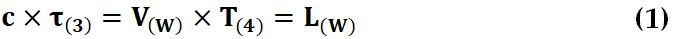Parameter c is a constant in the equation.

4. Let's study the simplest case, all four-dimensional objects travel at a same speed V(4).

### Object's event time dilation effect in three-dimensional real space

As Figure 2, there are two objects O41, O42. O41 and O42 travel in virtual abstract four-dimensional Euclidean geometry space at same speed but different direction. The direction of O41 is perpendicular to X-axis, Y-axis, and Z-axis. The direction of O42 is perpendicular to Y-axis, Z-axis but is not perpendicular to X-axis.

Figure 2. Two objects travel in a virtual abstract four-dimensional Euclidean geometry space at same speed but different direction

According to the procedure 4, in one unit time of virtual abstract four-dimensional Euclidean space, the distance that O41 and O42 travel is: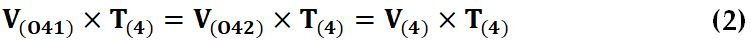According to the procedure 3, within the three-dimensional real space, O41 is static, the velocity of O41 all contribute to velocity in W-axis direction, it mean all the velocity of O41 have translate into object's event time in the three-dimensional real space, we can regard the event time in O41 reference frame as the standard time in three-dimensional real space. The velocity of O42 contributes to both velocity in W-axis direction and velocity in X-axis. The velocity along the X-axis is object motion in the three-dimensional real space, the velocity along the W-axis is the object's event time in the three-dimensional real space.

According to the Pythagorean Theorem:

Equation (3) same as: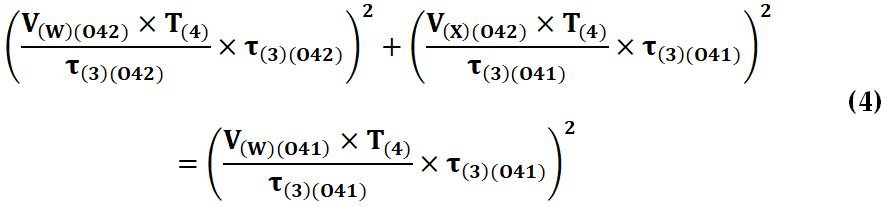Within the three-dimensional real space, In one unit time of the virtual four-dimensional Euclidean geometry space T(4), the event time length τ for O41 and O42 is: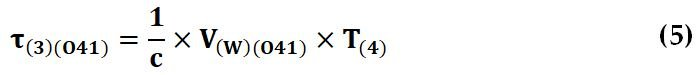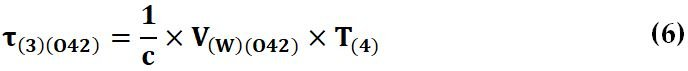From Equation (4), Equation (5), Equation (6), we have: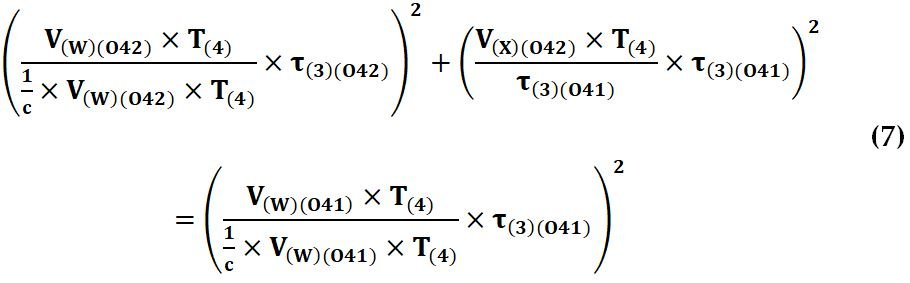Set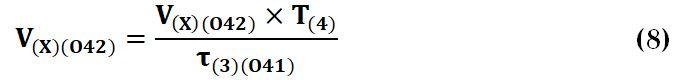to be the absolute velocity of O42 and Simplifying Equation (7), we have: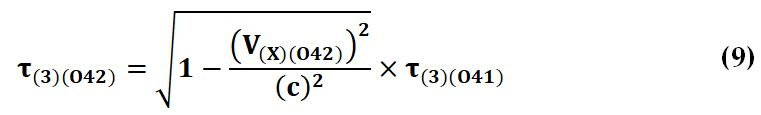### Length contraction effect in three-dimensional real space

According to the procedure 3, the perpendicular projections length of O42 to X-axis is relate to the direction of O42 travel in virtual abstract four-dimensional Euclidean space.

Figure 3. If O42 travel in a direction no perpendicular to X-axis, the original length AB of O42 will contract to length of AC in three-dimensional real space

As figure 3, the accelerated motion of four dimensional objects will cause the object to contract in X-axis around the center of moment, the relation between the length of O42 in virtual abstract four-dimensional Euclidean space and the length of O42 perpendicular projections to X-axis is:

As: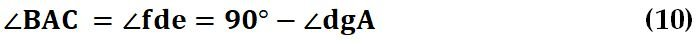We have: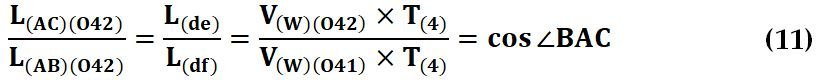From Equation (5), Equation (6), we have: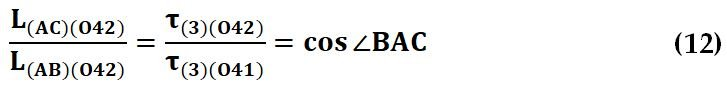From Equation (9), Equation (12), we have: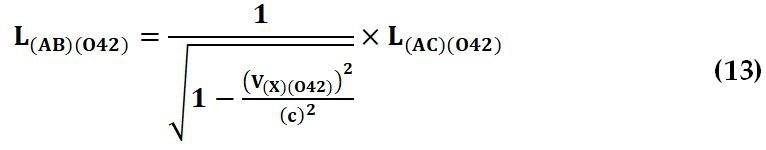### A maximum speed

If a four-dimensional object G travels in a direction perpendicular to the W dimension. Within the three-dimensional real space, the absolute speed of G is: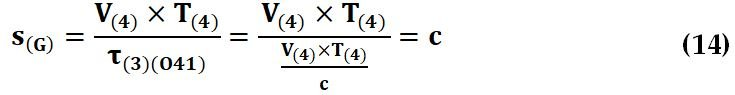This is the maximum speed in three-dimensional real space.

### The postulate of constancy of the speed of light and the time dilation/length contraction effect are two independent conclusions

It can be seen that the geometric model of time dilation/length contraction has ensured that the speed of light is the maximum speed in the universe and parameter c is a constant when calculating time dilation effect and length contraction effect. At the same time, it can be seen that time dilation/length contraction effect does not have any relation with the postulate of constancy of the speed of light. They are actually two independent conclusions. Time dilation/length contraction effect does not prove the postulate of constancy of the speed of light; at the same time, it is impossible to deduce the time dilation/length contraction effect from the postulate of constancy of the speed of light in strict logic mode.

Condition for the speed of light to be constant:
The constant speed of light is a derivation of Special relativity velocity addition formula, The Special relativity velocity addition formula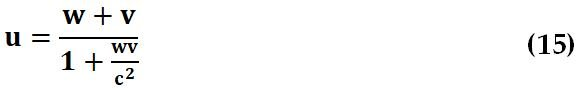Is obtained by dividing the x' by the t' from Lorentz Transformation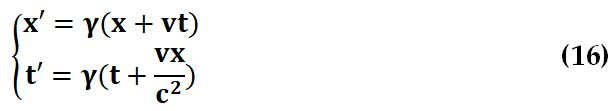Where γ=1/((1-vv/cc)^1/2)
Since dividing the x' by the t' will eliminate the γ, the γ value is not a necessary condition for the speed of light to be constant. The factor vx/cc is the sufficient condition for the postulate of constancy of the speed of light. We can derive the Special relativity velocity addition formula when γ=1 too.

When γ=1, the coordinate transformation is: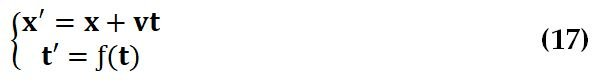Presume that the speed of light is constant, we have: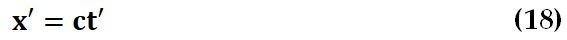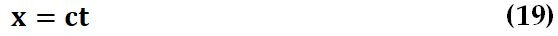Replace x' with ct' and x with ct in equation 17, we have: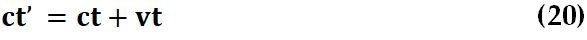To make the above formula hold:

Method 1 is to let t' = ((c+v)/c)t. equation 17 will be transformed into equation 21: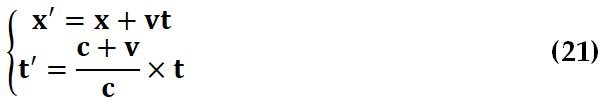Method 2 is since ct=x, so we can convert vt to vx/c, equation 17 will be transformed into equation 22.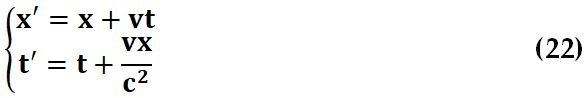Both equation 21 and equation 22 correspond to postulate of constancy of the speed of light, but does not predict time dilation/length contraction effect at all.

A coordinate transformation that describe time dilation/length contraction effect, but without postulate of constant speed of light is: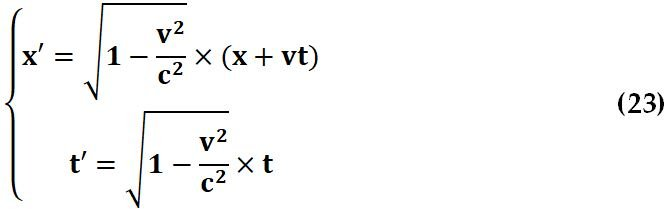Where c is constant and v<=c.

If we take into account that time dilation/length contraction effect isn't evidence for postulate of constancy of the speed of light, there is no sufficient experimental evidence for the postulate of constancy of the speed of light. We need more experiments to verify the postulate of constancy of the speed of light, like:
1. Measure one-way speed of light (please sees the result of E. D. Greaves's one-way speed of light experiment and reconsiders the results of the Doppler effect of light).
2. Comparing the speed difference between two lights going back and forth (which have been measured in Sagnac effect, In particular, Wang Ruyong's linear Sagnac experiment indicates that there is Sagnac effect in inertial motion, LinJin's two-way satellite time and frequency transfer experiment indicates that there is Sagnac effect in the orbital motion of artificial satellites).
3. Whether the vx/cc factor can be measured in time dilation experiments. In special relativity, we ignore the vx/cc factor when we deduce the time dilation formula. When x=ct, vx/cc is equivalent to vt/c. If the results of experiments exactly match t'=γt, it just means that the vx/cc factor should be excluded from the Lorentz transformation.
4. Comparing the velocity addition of special relativity with the velocity addition of this model. There is only one relativistic velocity addition formula in special relativity. In this model, the velocity addition is divided into two types:
A. The addition of the initial velocity and secondary velocity of an object
I. Convert the velocity measured in the inertial frame into a standard secondary velocity according to the time dilation/length contraction effect.
II. Suppose the initial velocity is v(1), the standard secondary velocity is v(2), the angle between the initial velocity and the standard secondary velocity is θ, the addition velocity v(3) of v(1) and v(2) is:

Where v(3)≤c
Suppose that the secondary velocity measured in the inertial system is v(2)' and the angle between the initial velocity and the secondary velocity measured in the inertial system is θ', then:

When θ' is 90°, we get an interesting special case:

Equation 26 is similar to the special relativity velocity addition formula. When v(1) = c or when v(2)' = c, the addition velocity of v(1) and v(2)' is also c.
When θ' = 0° and θ' = 180°, the maximum velocities (the speed of light) measured in the v(1) reference frame are c-v(1) and c+v(1), this will predicts Sagnac effect from the v(1) reference frame.
B. Addition of absolute velocities of two objects.
Add the absolute velocities of the two objects using the principle of vector addition, divide by the length of time of the observer's reference frame.
In absolute real space, the velocities of the two objects conform to the principle of vector addition, that is, the velocities of the observer and the speed of light going back and forth are c-v and c+v, this will predicts Sagnac effect from the perspective of absolute real space.

### Other evidence that objects maybe four spatial dimensional

In this section, I would like to show you that this model is compatible with general relativity too. In this model, real space consists of three-dimensional physical entities, and object consists of four-dimensional physical entities. Evidence for this conclusion can be found in quantum mechanics and classical mechanics.

1. Evidence from quantum mechanics
Since objects have properties of four dimensions, physical space have properties of three dimensions, we could conclude that:
A. Four-dimensional object is superposition of multiple three-dimensional states;
B. In three dimensional physical space, only one three dimensional state of object is detected in physical event.
These conclusions is relate to the quantum superposition and quantum collapse in quantum mechanics.

2. Evidence from classical mechanics
If the four-dimensional volume of the four-dimensional object is taken as the static inertial mass of the object in the three-dimensional physical space, when the four-dimensional object pushes away one unit of the three-dimensional physical entity, the energy consumed by the four-dimensional object is: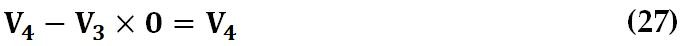That mean four-dimensional objects lost zero energy and will continues to move at a constant velocity in three-dimensional physical space. This conclusion relate to Newton’s first law. At the same time, we can see that relativistic mass of object is a leverage effect from this model.

## Conclusions

This article proposed how to define abstract space and instantiate physical entities, which provide a new insight into the interaction between four-dimensional objects and three-dimensional space. And then introduce a geometric model that has a maximum speed. It shows that the geometric model of time dilation/length contraction has ensured that the speed of light is the maximum speed in the universe, and since experiments do not support the postulate of constant speed of light, the vc/cc factor in the Lorentz transformation does not correspond with reality and should be excluded from the Lorentz transformation. After converting the local velocity according to the time dilation/length contraction effect to standard velocity, the standard velocity still conforms to the principle of vector addition.

## References

 Einstein A, Zur Elektrodynamik bewegter Körper, Annalen der Physik, 17, 891, 1905
 J. M. C. Montanus, Special relativity in an absolute Euclidean Space-Time, Phys. Essays, vol 4, nr 3, 1991
 Alexander Gersten, Euclidean Special Relativity , Found. Phys. 33, 2003, Pages 1237-1251
 Wang R., From the Triangle Sagnac Experiment to a Practical Crucial Experiment of the Constancy of the Speed of Light Using Atomic Clocks on Moving Objects, Europhysics Letters, 1998, 43(6): 6112616
 E. D. Greaves, A. M. Rodriguez, and J. Ruiz-Camacho, A one-way speed of light experiment, Am. J. Phys.77, 894 (2009)
 LinJin, Li Zhi-gang, Fei Jing-gao, HU De-feng, The crucial experiment for checking Einstein's postulate of the constancy of the speed of light, Journal of Astronautics, 2009, vol 30: 25-32
 Yuan­Zhong Zhang, Special Relativity and Its Experimental Foundations, World Scientific Pub Co Inc, 1997, ISBN 978-981-02-2749-4
 Wen-Hung Tseng, Kai-Ming Feng, Shinn-Yan Lin, Huang-Tien Lin, Yi-Jiun Huang, Chia-Shu Liao, Sagnac effect and diurnal correction on two-way satellite time transfer, IEEE Trans. Instrum. Meas., vol. 60, no. 7, pp. 2298-2303, Jul. 2011.

##### Share on other sites

I have not yet had the time to study the detail of your proposal but a couple of points which I would like clarified stand out.

1.  Did I just mention time ? You appear to be introducing a fourth spatial dimension. What does that do to using 'ct' in modern treatments of SR ?
I note your statement that Montanus studied the effect of different combinations and numbers of both spatial and temporat dimensions.
A good development of the mathematics of what happens and why we choose the option we have appears in Eddington's book on the subject.

2. I suppose it depends upon your meaning of the word 'classical' but I cannot agree that:

54 minutes ago, mythxp said:

There is no definition of abstract dimensions in classical geometry,

You will find a full treatment in for instance Sommerville's "An Introduction to The geometry of N dimensions".
There is lots more particularly if you move to what is known as 'algebraic geometry'

By all means propose that the physical world does not conform to our abstract mathematics - There are. after all, well  known differences.
But then you cannot (directly) apply the theorems and results of this mathematics to your proposals, you will need to develop your own.

##### Share on other sites

A couple more points have occurred to me on further reading.

3.

3 hours ago, mythxp said:

2: According to the reasoning A, four-dimensional object should be isotropic (connect itself end to end) in the fourth abstract dimension if there is only a three dimensional real space, four-dimensional object cannot move out of the three dimensional real space but always have an intersection point with three dimensional real space.

You can embed an object of lower dimensionality in a space of N dimensions.

So you can have line in 2, 3 or more dimensions and it is still a line.

But you cannot do it the other way round. A 3D object will not fit into a space of only 2 dimensions.

Now I see what you are saying about a 4D object 'passing through'    however that appears to me to contain a logical inconsistency.

In order to have a 4D object and for it to move so that different 3D sections appear in the 3D space you must also have 4 dimensions.

4.

You appear to have 'fitted' you equations to observation with no foundational justification.

It is a fact that I coulod take any bunch of (random) points on a graph and fit an infinite count of different curves passing through those points.

You need the foundational justification as to why Nature selects your particular equation set over any other.

##### Share on other sites

3 hours ago, mythxp said:

Condition for the speed of light to be constant:
The constant speed of light is a derivation of Special relativity velocity addition formula, The Special relativity velocity addition formula

Is obtained by dividing the x' by the t' from Lorentz Transformation

Where γ=1/((1-vv/cc)^1/2)
Since dividing the x' by the t' will eliminate the γ, the γ value is not a necessary condition for the speed of light to be constant.

The velocity addition formula is not the reason that relativity says light speed is invariant, it's a result of it. And you haven't actually derived the formula here.

##### Share on other sites

• 2 weeks later...
On 7/19/2022 at 6:29 PM, studiot said:

A couple more points have occurred to me on further reading.

3.

You can embed an object of lower dimensionality in a space of N dimensions.

So you can have line in 2, 3 or more dimensions and it is still a line.

But you cannot do it the other way round. A 3D object will not fit into a space of only 2 dimensions.

Now I see what you are saying about a 4D object 'passing through'    however that appears to me to contain a logical inconsistency.

In order to have a 4D object and for it to move so that different 3D sections appear in the 3D space you must also have 4 dimensions.

4.

You appear to have 'fitted' you equations to observation with no foundational justification.

It is a fact that I coulod take any bunch of (random) points on a graph and fit an infinite count of different curves passing through those points.

You need the foundational justification as to why Nature selects your particular equation set over any other.

Object and real space are two real physical entities, they are different in the dimension. Object is a physical entity of four dimensions, and real space is a physical entity of three dimensions. We can view object to be the real four-dimensional space itself, we don't need a four-dimensional real space to hold object, we need an abstract (non-existent) (mathematical)(reset to 0 if not instantiated by a physics entity) geometric space to describe four-dimensional object.

On 7/19/2022 at 6:33 PM, swansont said:

The velocity addition formula is not the reason that relativity says light speed is invariant, it's a result of it. And you haven't actually derived the formula here.

you could see the derivation process in the link below.
https://newt.phys.unsw.edu.au/einsteinlight/jw/module4_Lorentz_transforms.htm
You said that u=(w+v)/(1+wv/cc) is the result of light speed is invariant , but formula 24 in my article conform to the postulate of constant speed of light too, there should by many addition formulas that conform to the postulate of constant speed of light, are all these velocity addition formulas the result of light speed is invariant?

On 7/19/2022 at 6:33 PM, swansont said:

The velocity addition formula is not the reason that relativity says light speed is invariant, it's a result of it. And you haven't actually derived the formula here.

I just mean that: in special relativity, the only mathematical expression of the postulate of constant speed of light is u=(w+v)/(1+wv/cc)

##### Share on other sites

2 hours ago, mythxp said:

Object and real space are two real physical entities, they are different in the dimension. Object is a physical entity of four dimensions, and real space is a physical entity of three dimensions. We can view object to be the real four-dimensional space itself, we don't need a four-dimensional real space to hold object, we need an abstract (non-existent) (mathematical)(reset to 0 if not instantiated by a physics entity) geometric space to describe four-dimensional object.

I went to the time and trouble of carefully reading and analysing you longish initial posting.
Then I asked you only 4 very clear questions about

It has taken you two weeks to not answer even one of them.

The above short reply was a repetition of what you already wrote and not a response to my question about that particular point.

I'm sorry I wasted my time.

##### Share on other sites

3 hours ago, mythxp said:

you could see the derivation process in the link below.
https://newt.phys.unsw.edu.au/einsteinlight/jw/module4_Lorentz_transforms.htm
You said that u=(w+v)/(1+wv/cc) is the result of light speed is invariant , but formula 24 in my article conform to the postulate of constant speed of light too, there should by many addition formulas that conform to the postulate of constant speed of light, are all these velocity addition formulas the result of light speed is invariant?

In Galilean relativity, velocity addition is linear.

3 hours ago, mythxp said:

I just mean that: in special relativity, the only mathematical expression of the postulate of constant speed of light is u=(w+v)/(1+wv/cc)

Only? There are, quite notably, the Lorentz transforms.

##### Share on other sites

13 hours ago, mythxp said:

the only mathematical expression of the postulate of constant speed of light is u=(w+v)/(1+wv/cc)

The speed of light is invariant, not constant. That’s an important difference. Furthermore then it is directly related to the electromagnetic properties of the underlying medium:

$c=\frac{1}{\sqrt{\epsilon_{0} \mu_{0}}}$

Since these are fundamental constants, irrespective of anyone’s state of motion, c has to have the same numerical value in all reference frames.

##### Share on other sites

• 1 month later...

I have already explained why c is a constant in Equation 1, don't judge without reading anything, OK

Attach a mind map to help you understand my model

On 7/30/2022 at 4:24 AM, Markus Hanke said:

The speed of light is invariant, not constant. That’s an important difference. Furthermore then it is directly related to the electromagnetic properties of the underlying medium:

c=1ϵ0μ0

Since these are fundamental constants, irrespective of anyone’s state of motion, c has to have the same numerical value in all reference frames.

Can you tell me what is the difference between invariant and constant? Is gravity the same everywhere because of there is Gravitational Constant G ?

On 7/29/2022 at 6:15 PM, swansont said:

Only? There are, quite notably, the Lorentz transforms.

Please provide another formula for the constant speed of light instead of  (u+v)/(1+uv/cc),  how the Lorentz transformation you mentioned proves that the speed of light is constant

Edited by mythxp
##### Share on other sites

4 hours ago, mythxp said:

Please provide another formula for the constant speed of light instead of  (u+v)/(1+uv/cc),  how the Lorentz transformation you mentioned proves that the speed of light is constant

You claimed that this was "the only mathematical expression of the postulate of constant speed of light"

The Lorentz transforms are derived in Einstein's 1905 paper, based on the invariant speed of light. They are an expression of that postulate. As is his later derivation of E=mc2

Try deriving these equations without c being invariant! That might not be proof of c being invariant, but I never claimed anything about proof, only that they are mathematical expression of it. (Though one could probably do a proof by contradiction)

##### Share on other sites

On 9/22/2022 at 5:34 PM, mythxp said:

Can you tell me what is the difference between invariant and constant?

Constant means it has the same numerical value everywhere, whereas invariant means that it doesn’t change when going into another reference frame.

The speed of light depends on the medium, so it’s value is always invariant, but constant only within the same medium.

On 9/22/2022 at 5:34 PM, mythxp said:

Is gravity the same everywhere

I don’t know what you mean by this...? It’s obviously not the same everywhere, since it depends on the distribution of sources.

On 9/22/2022 at 5:34 PM, mythxp said:

Please provide another formula for the constant speed of light instead of  (u+v)/(1+uv/cc)

$c=\frac{1}{\sqrt{\mu_{0} \epsilon_{0}}}$

##### Share on other sites

3 hours ago, Markus Hanke said:

The speed of light depends on the medium, so it’s value is always invariant, but constant only within the same medium.

Let's not muddle things. We're talking about c, the speed of light in a vacuum, which means there is no medium. That value is constant and invariant.

## Create an account or sign in to comment

You need to be a member in order to leave a comment

## Create an account

Sign up for a new account in our community. It's easy!

Register a new account